## Velocity

Subject: Science

#### Overview

The velocity of the body is the distance travelled by it in unit time to a certain direction. If a moving body covers the equal distance in equal interval of time , then the body is said to have uniform velocity. If a moving body covers the different distance in unit time then the body is said to have variable velocity. This note provides information about velocity, definition, example and help for numerical problem.
##### VelocityThe velocity of the body is the distance travelled by it in unit time in a certain direction. It is the rate of change of the distance of an object in a particular direction.If a body travels a distance of S-meter in t seconds in a given direction, then,

Velocity = Distance travelled in a given direction/ time taken=S/T (meters per second)

#### Average Velocity

Average velocity can be defined as the distance travelled divided by the time elapsed.

average velocity= displacement/time taken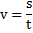here, v= velocity

s= displacement

t= time taken

Example: If a car travels 10 meters in 2 seconds and travels 32 meters in another 5 seconds then calculate its' average velocity.

Solution here,

distance (d1) = 10 m

distance (d2) = 32 m

time (t1) = 2 s

time (t2) = 5 s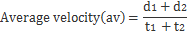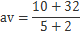= 42/7 = 6 m/s.

Therefore, the average velocity is m/s.

If initial velocity of an object is u and final velocity of an object is v then,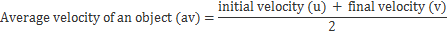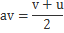Example: If a tempo travels with a speed of 5 m/s. How far will it reach in one more hour?

Here,

Speed (v) = 5 m/s

Time (t) = 1 hour

= 60 x 60 sec

= 3600 sec

Distance(s) =?

We have,Or, s = v x t

= 5 m/s x 3600 s

= 18000 m

= 18 km

Thus, the tempo travels 18 km in one hour.

The unit of velocity is the same as that of speed, namely, meter per second. Velocity has magnitude as well as direction, therefore, velocity is a vector quantity.The velocity of a car shows its definite direction.• Uniform velocity: In uniform velocity, a moving body covers the equal distance in equal interval of time, then the body is said to have uniform velocity.
• Variable velocity: If a moving body covers the different distance in unit time then the body is said to have variable velocity.
• Relative velocity: The laws of physics which apply when you are at rest on the earth also apply when you are in any reference frame which is moving at a constant velocity with respect to the earth. The motion may have the different appearance as viewed from a different reference frame, but this can be explained by relative point.

For example, People in running vehicle is at rest with respect to the vehicle but at motion with respect to the road.

##### Things to remember
• The velocity of the body is the distance travelled by it in unit time to a certain direction.
• Uniform velocity: If a moving body cover the equal distance in equal interval of time , then the body is said to have uniform velocity.

• Variable velocity:If a moving body covers  the different distance in unit time then the body is said to have variable velocity.

• Relative velocity: The laws of physics which apply when you are at rest on the earth also apply when you are in any reference frame which is moving at a constant velocity with respect to the earth.

• It includes every relationship which established among the people.
• There can be more than one community in a society. Community smaller than society.
• It is a network of social relationships which cannot see or touched.
• common interests and common objectives are not necessary for society.
##### Questions and Answers

If a moving body covers equal distances in equal intervals of time, then the body is said to have uniform velocity.

The S.I unit of velocity is meter per second.

When a body is moving in a curved path with uniform speed, it’s speed remains same but the velocity is changing at each point of the curved path. This is because the direction of motions of the body is changing continuously.

Light travels 300000km/s is a velocity because light travels in a straight line in a fixed path.

Solution:
Initial velocity of a car = 72 k/hr
= (72 x 1000/60 x 60)
= 72000/300
= 20 m/s
Final velocity of truck = 0
Time taken = 5 sec
Distance =?
We know,
a = v – u / t
= 0 – 20/ 5
= -20/5
= -4 m/s²
Now, distance (s) = u x t + ½ at²
= 20 x 5 + ½ (-4) x 5²
= 100 - ½ x 4 x 25
= 100 - ½ x 50
= 100 – 50
= 50 m

Solution,
Initial velocity (u) = 20 m/s
Final velocity (v) = 0
Time taken (t) = 2 sec
Height (h) =?
Acceleration due to gravity = 10m/s²
We know,
v² = u² - 2gh
or, 0² = 20 – 2 x 10 x h
or, 0 = 400 – 20h
or, 20h = 400 – 0
or, h = 400/20
h = 20 m
Thus, the height after 2sec. is 2m.